# Solution assignment 10 Unit circle and simple formulas

### Assignment 10

Show for which values ofin the interval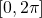the function: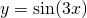takes the value.

### Solution

The function has three periods in the given interval. In one period the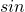-functie takes the valueonly once. So this will happen three times in the given interval of: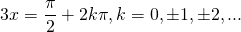This yields the following values in the given interval: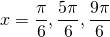0